International
Tables for
Crystallography
Volume B
Reciprocal space
Edited by U. Shmueli

International Tables for Crystallography (2010). Vol. B, ch. 2.5, pp. 349-352   | 1 | 2 |

## Section 2.5.3.4.3. Space-group determination

M. Tanakaf

#### 2.5.3.4.3. Space-group determination

| top | pdf |

Table 2.5.3.13shows the space-group symbols (fourth column) of the modulated crystals. When a glide (screw) component τ4 between the modulation waves of two atom rows is 0, 1/2, ±1/3, ±1/4 or ±1/6, symbol 1, s, t, q or h is given, respectively (de Wolff et al., 1981). Such glide components are allowed for point-group symmetry 1 but are not for point-group symmetry. Dynamical extinction occurs for glide components s, q and h but does not for glide component t. When the average structure does not have a glide component, dynamical extinction due to a glide component τ4 appears in odd-order incommensurate reflections. When the average structure has a glide component, dynamical extinction due to a glide component τ4 appears in incommensurate reflections with hi + h4 = 2n + 1, where hi and h4 are the reflection indices for the average structure and incommensurate structure, respectively. Details are given in the paper by Terauchi et al. (1994).

Fig. 2.5.3.20(a) illustrates mirror symmetry () between atom rows A and B, which is perpendicular to the b axis with no glide component (τ4 = 0). Here, the wave number vector of the modulation is assumed to be k = k3c* following the treatment of de Wolff et al. (1981). Fig. 2.5.3.20(b) illustrates glide symmetry () with a glide component τ4 = ½. The structure factor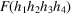is written for the glide plane () of an infinite incommensurate crystal as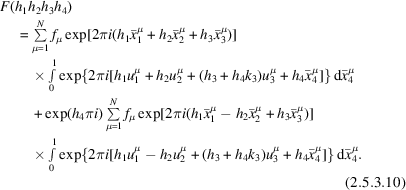Thus, the following phase relations are obtained between the two structure factors: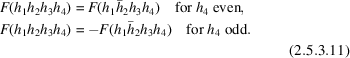These relations are analogous to the phase relations between the two structure factors for an ordinary three-dimensional crystal with a glide plane. The relations imply that dynamical extinction occurs for the glide planes and screw axes of the (3 + 1)-dimensional crystal with an infinite dimension along the direction of the incommensurate modulation wavevector k. Terauchi et al. (1994) showed that approximate dynamical extinction occurs for an incommensurate crystal of finite dimension.Figure 2.5.3.20 | top | pdf |(a) Mirror symmetry of modulation waves () τ4 = 0. (b) Glide symmetry of modulation waves () τ4 = ½. The wave number vector of modulation is k3c*.

Fig. 2.5.3.21(a) and (b) illustrate a spot diffraction pattern and a CBED pattern, respectively, expected from a modulated crystal with a (3 + 1)-dimensional space group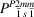(k = k3c*) at the  incidence. The large and small spots in Fig. 2.5.3.21(a) designate the fundamental (h4 = 0) and incommensurate reflections (h4 ≠ 0), respectively. The 00h3h4 (h4 = odd) reflections shown by crosses are kinematically forbidden by the glide plane () perpendicular to the b axis. Umweganregung paths a, b and c in the ZOLZ to a kinematically forbidden reflection are drawn. The two paths a and b are geometrically equivalent with respect to the line mm perpendicular to the b axis. Since every Umwegan­regung path to a kinematically forbidden reflection contains an odd number of F(0h2,ih3,ih4,i) with odd h4,i, the following equation is obtained.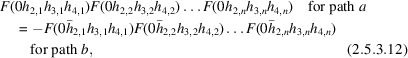where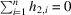,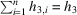and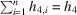(h4 = odd).Figure 2.5.3.21 | top | pdf |(a) Umweganregung paths a, b and c to the 0011 forbidden reflection. (b) Expected dynamical extinction lines are shown, the 0011 reflection being excited. The wave number vector of modulation is k3c*.

When reflection 00h3h4 (h4 = odd) is exactly excited, the two paths a and c are symmetric with respect to the bisector m′–m′ of the diffraction vector of the reflection and have the same excitation error. The waves passing through these paths have the same amplitude but different signs. Thus the following relation is obtained.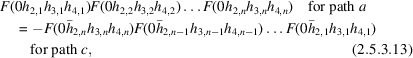where,and(h4 = odd).

Therefore, dynamical extinction occurs in kinematically forbidden reflections of incommensurate crystals. Fig. 2.5.3.21(b) schematically shows the extinction lines in odd-order incommensurate reflections, where the 0011 reflection is exactly excited.

We consider the dynamical extinction from Sr2Nb2O7 whose space group is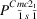. The glide plane () is perpendicular to the b axis with a glide vector (c + a4)/2. The wave number vector of the modulation is k = (½ − δ)a*. (Since space-group notation Cmc21 is broadly accepted, the direction of the modulation is taken as the a axis.) The reflections h10h3h4 with h3 + h4 = 2n + 1 (n = integer) are kinematically forbidden. Fig. 2.5.3.22shows a schematic diffraction pattern of Sr2Nb2O7 at the  incidence. The large and small spots indicate the fundamental (h4 = 0) and incommensurate (h4 ≠ 0) reflections, respectively. Umweganregung paths a and b to the kinematically forbidden 0001 reflection via a fundamental reflection in the ZOLZ are drawn.Figure 2.5.3.22 | top | pdf |Schematic diffraction pattern at the  incidence of Sr2Nb2O7. Umweganregung paths a and b via fundamental reflections to the 0001 incommensurate reflection. Large and small spots denote fundamental and incommensurate reflections, respectively. The wave number vector of modulation is k = (½ − δ)a*.

Fig. 2.5.3.23(a) shows a spot diffraction pattern of the incommensurate phase of Sr2Nb2O7 taken with the  incidence at 60 kV. The incommensurate reflections in which dynamical extinction lines appear at this incidence are those with the indices h1,even00h4,odd because h3 = 0 and h1 + h2 = 2n due to the lattice type C of the average structure.Figure 2.5.3.23 | top | pdf |Diffraction pattern of Sr2Nb2O7 taken with  incidence at 60 kV. (a) Spot diffraction pattern. Kinematically forbidden 0001 and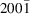incommensurate reflections exhibit definite intensity. (b) Zone-axis CBED pattern showing dynamical absence of 0001 andincommensurate reflections. (c) CBED pattern taken at an incidence with a small tilt from the zone axis to the b* direction. The kinematically forbidden incommensurate reflections have intensity due to incomplete cancellation of two waves through the Umweganregung paths. The wave number vector of modulation is k = (½ − δ)a*.

The reflections in the four columns indicated by black arrowheads are incommensurate reflections. The reflections 0001,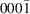,and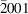designated by white arrowheads are kinematically forbidden but exhibit certain intensities, which are caused by multiple diffraction. Other reflections are fundamental reflections due to the average structure.

Fig. 2.5.3.23(b) shows a CBED pattern corresponding to Fig. 2.5.3.23(a), taken from a specimen area 3 nm in diameter. The excitation errors of two Umweganregung paths a and b are the same at this electron incidence. The reflections 0001,,andindicated by white arrowheads show no intensity. Dynamical extinction does not appear as a line in the present case because the width of the extinction line exceeds the disc size of the reflections. Fig. 2.5.3.23(c) shows a CBED pattern taken at an incidence slightly tilted toward the b* axis from that for Fig. 2.5.3.23(b) or the  zone-axis incidence. The excitation errors are no longer the same for the two Umweganregung paths. Thus, it is seen that the kinematically forbidden reflections indicated by white arrowheads have intensities due to incomplete cancellation of waves coming through different paths, which is an additional proof of the dynamical extinction.

### References

Terauchi, M., Takahashi, M. & Tanaka, M. (1994). Convergent-beam electron diffraction study of incommensurately modulated crystals. II. (3+1)-dimensional space groups. Acta Cryst. A50, 566–574.
Wolff, P. M. de, Janssen, T. & Janner, A. (1981). The superspace groups for incommensurate crystal structures with a one-dimensional modulation. Acta Cryst. A37, 625–636.